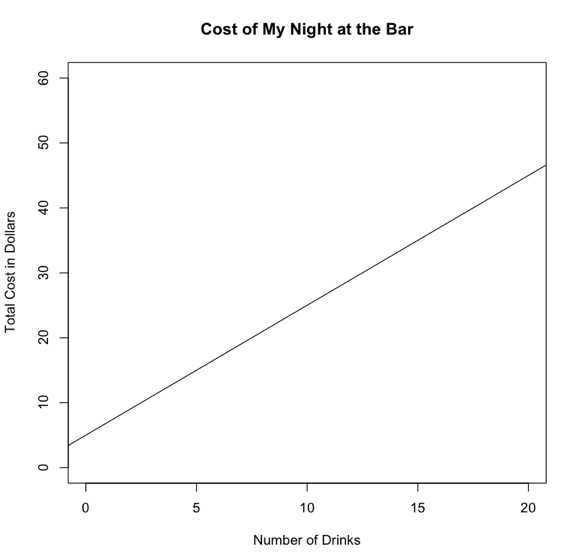When the value of one variable is plotted against the value of another variable, and the plotted points fall on a straight line, the relationship between the two variables is called a linear relationship. Linear relationships are additive relationships, which is to say, as the value of one variable changes by a constant amount, the value of the second variable also changes by a constant amount. For example, you pay a \$5 cover charge to get into a bar, and then you pay \$2 per drink thereafter. Every time you increase the number of drinks you've had by one, the amount of money that has left your pocket increases by \$2. The total cost of your evening in the bar will be: cost = 5 + 2 * number of drinks This is an example of a linear equation. The general form of a linear equation is: y = a + bx The letters a and b are entirely arbitrary. Any other letters can be substituted for them, and you just have to see the book you are using to see what form the author is using for linear equations. For example, most introductory algebra books use: y = mx + b (It also doesn't matter if the part with the x comes first or second.) In statistics we often write: y = b0 + b1x The point is there will be a letter standing by itself, and there will be a letter accompanied by an x (or whatever symbol is being used for the variable). Both of these values have meaning. The letter that stands by itself is called the y-intercept of the linear function. The letter that stands with the x is called the slope. These letters, which stand for constant values, are called coefficients. To use the example of your night in the bar, if we let y stand for your total cost, and we let x stand for the number of drinks you've had, then: y = 5 + 2x A graph of this equation would look like this. The line shows how much you would pay depending upon how many drinks you've had.(By this time I suspect they'd be calling you a cab!) In linear equations of the form y = a + bx, x is often referred to as the independent variable, and y is referred to as the dependent variable . The reason is simple. y is stated as a function of x, so the value that y gets depends upon the value that x gets. More formally, we say that y is a function of x, or y = f(x), which is to say, the dependent variable is a function of the independent variable. In fact, to be completely correct, the relationships we are discussing here would be called linear functions rather than linear equations, but in statistics we usually refer to them as linear equations. The fact that x and y are referred to as variables simply means that their values can change, or vary. We have IVs and DVs in statistics, too, and the meaning of those terms to a statistician is not much different than they are here. The value that we record for the DV from any given subject depends upon the subject's behavior. We like to think that the subject's behavior, in turn, depends upon the value of the IV that we as experimenters expose him or her to. In a true (or designed) experiment, the subject has no control over the value of the IV he is exposed to. It does not depend on him. It's independent of his behavior. In a linear equation, the coefficient of x defines the slope of the line, or how tilty it is. Slope is sometimes defined as "rise over the run," or how far the line rises (or falls, basically the change in the y direction) for every unit of run (change in the x direction). In more formal terms, slope is: b = (y2 - y1) / (x2 - x1) The slope may be positive, in which case the line slants from the lower left towards the upper right of the graph (as above). It may be negative, in which case the line slants downward from the upper left to the lower right. Or it may be zero, in which case the line is horizontal. Lines with the same slope are parallel. Return To Main Page